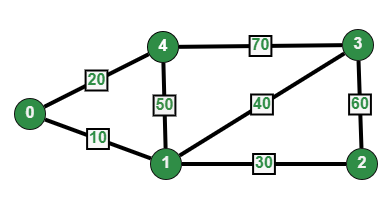# Graph implementation using STL for competitive programming | Set 2 (Weighted graph)

• Difficulty Level : Easy
• Last Updated : 28 Apr, 2022

In Set 1, unweighted graph is discussed. In this post, weighted graph representation using STL is discussed. The implementation is for adjacency list representation of weighted graph.Undirected Weighted Graph

We use two STL containers to represent graph:

• vector : A sequence container. Here we use it to store adjacency lists of all vertices. We use vertex number as index in this vector.
• pair : A simple container to store pair of elements. Here we use it to store adjacent vertex number and weight of edge connecting to the adjacent.

The idea is to use a vector of pair vectors. Below code implements the same.

## C++

 `// C++ program to represent undirected and weighted graph``// using STL. The program basically prints adjacency list``// representation of graph``#include ``using` `namespace` `std;` `// To add an edge``void` `addEdge(vector > adj[], ``int` `u,``                                     ``int` `v, ``int` `wt)``{``    ``adj[u].push_back(make_pair(v, wt));``    ``adj[v].push_back(make_pair(u, wt));``}` `// Print adjacency list representation of graph``void` `printGraph(vector > adj[], ``int` `V)``{``    ``int` `v, w;``    ``for` `(``int` `u = 0; u < V; u++)``    ``{``        ``cout << ``"Node "` `<< u << ``" makes an edge with \n"``;``        ``for` `(``auto` `it = adj[u].begin(); it!=adj[u].end(); it++)``        ``{``            ``v = it->first;``            ``w = it->second;``            ``cout << ``"\tNode "` `<< v << ``" with edge weight ="``                 ``<< w << ``"\n"``;``        ``}``        ``cout << ``"\n"``;``    ``}``}` `// Driver code``int` `main()``{``    ``int` `V = 5;``    ``vector > adj[V];``    ``addEdge(adj, 0, 1, 10);``    ``addEdge(adj, 0, 4, 20);``    ``addEdge(adj, 1, 2, 30);``    ``addEdge(adj, 1, 3, 40);``    ``addEdge(adj, 1, 4, 50);``    ``addEdge(adj, 2, 3, 60);``    ``addEdge(adj, 3, 4, 70);``    ``printGraph(adj, V);``    ``return` `0;``}`

## Python3

 `# Python3 program to represent undirected``# and weighted graph. The program basically``# prints adjacency list representation of graph` `# To add an edge``def` `addEdge(adj, u, v, wt):``    ` `    ``adj[u].append([v, wt])``    ``adj[v].append([u, wt])``    ``return` `adj` `# Print adjacency list representation of graph``def` `printGraph(adj, V):``    ` `    ``v, w ``=` `0``, ``0``    ``for` `u ``in` `range``(V):``        ``print``(``"Node"``, u, ``"makes an edge with"``)` `        ``for` `it ``in` `adj[u]:``            ``v ``=` `it[``0``]``            ``w ``=` `it[``1``]``            ``print``(``"\tNode"``, v, ``"with edge weight ="``, w)``            ` `        ``print``()` `# Driver code``if` `__name__ ``=``=` `'__main__'``:``    ` `    ``V ``=` `5``    ``adj ``=` `[[] ``for` `i ``in` `range``(V)]` `    ``adj ``=` `addEdge(adj, ``0``, ``1``, ``10``)``    ``adj ``=` `addEdge(adj, ``0``, ``4``, ``20``)``    ``adj ``=` `addEdge(adj, ``1``, ``2``, ``30``)``    ``adj ``=` `addEdge(adj, ``1``, ``3``, ``40``)``    ``adj ``=` `addEdge(adj, ``1``, ``4``, ``50``)``    ``adj ``=` `addEdge(adj, ``2``, ``3``, ``60``)``    ``adj ``=` `addEdge(adj, ``3``, ``4``, ``70``)` `    ``printGraph(adj, V)` `# This code is contributed by mohit kumar 29`

## Javascript

 ``

Output:

```Node 0 makes an edge with
Node 1 with edge weight =10
Node 4 with edge weight =20

Node 1 makes an edge with
Node 0 with edge weight =10
Node 2 with edge weight =30
Node 3 with edge weight =40
Node 4 with edge weight =50

Node 2 makes an edge with
Node 1 with edge weight =30
Node 3 with edge weight =60

Node 3 makes an edge with
Node 1 with edge weight =40
Node 2 with edge weight =60
Node 4 with edge weight =70

Node 4 makes an edge with
Node 0 with edge weight =20
Node 1 with edge weight =50
Node 3 with edge weight =70```

This article is contributed by Sahil Chhabra. and improved by Kunal Verma If you like GeeksforGeeks and would like to contribute, you can also write an article using write.geeksforgeeks.org or mail your article to review-team@geeksforgeeks.org. See your article appearing on the GeeksforGeeks main page and help other Geeks.